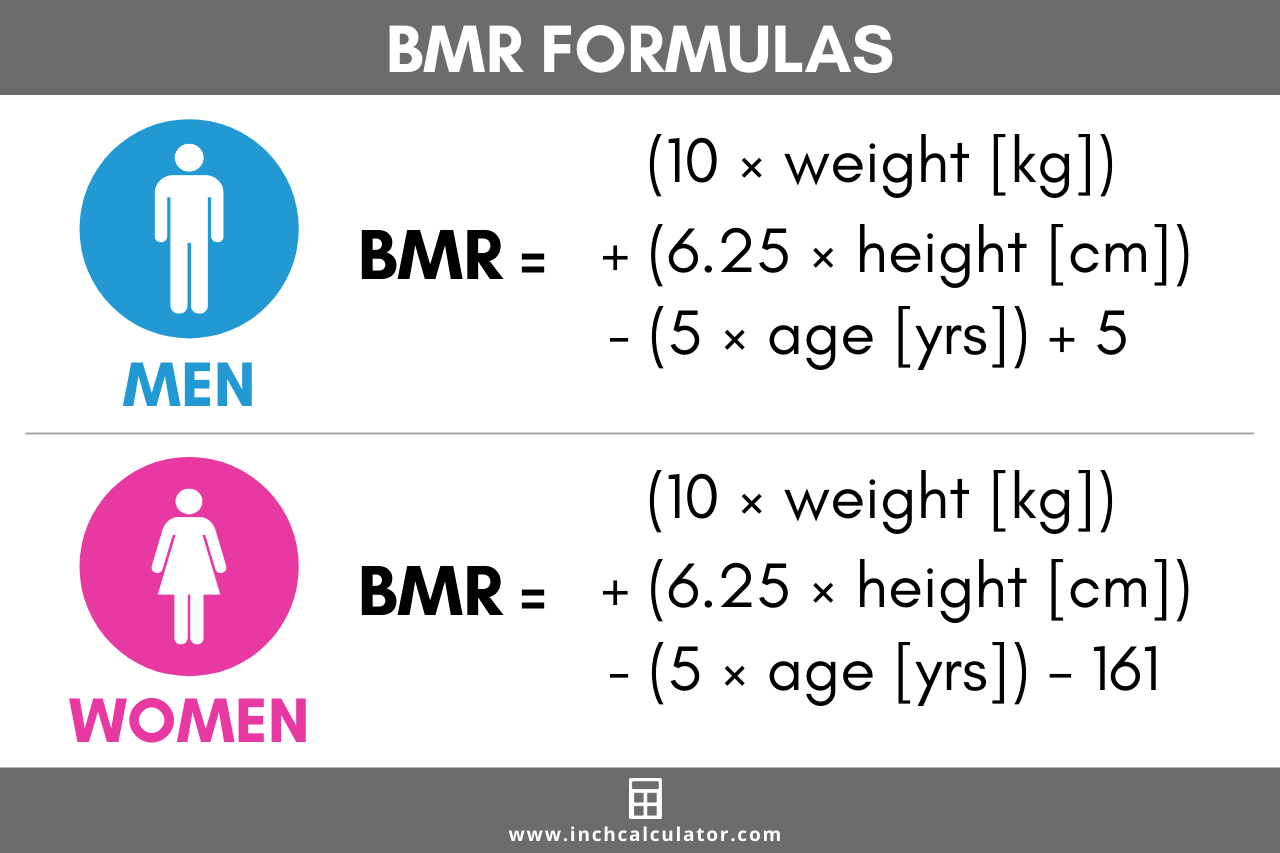# Mifflin St. Jeor Calculator (TDEE & BMR)

Calculate TDEE & BMR using the Mifflin St. Jeor equation.

Gender:
Gender:

## Your Total Daily Energy Expenditure (TDEE):

calories/day
Daily energy expenditures for various levels of activity.
Activity LevelCalories
Basal Metabolic Rate (BMR)
little to no exercise
light exercise 1-3 times per week
moderate exercise 3-5 times per week
heavy physical exercise 5-6 times per week
heavy physical exercise 6-7 times per week

For adults, a BMI in the range of 18.5 - 24.9 is considered healthy
Learn how we calculated this below

## How to Calculate BMR using the Mifflin St Jeor Equation

Basal metabolic rate is the amount of energy needed for your body’s physiological functions at rest, such as your breathing, your heart beating, and your brain activity.

This amount of energy required is measured in kilocalories, or often just denoted as calories. Therefore, BMR is your body’s calorie needs at rest with no extra activity.

In order to measure your exact BMR, you need to be in a lab setting and use indirect calorimetry. This measurement is taken under tightly controlled conditions and not easily accessible to most people.

Caloric expenditure measured by indirect calorimetry can then be added to physical activity energy expenditure to calculate total daily energy expenditure.

Since most people don’t have the ability to have indirect calorimetry performed, formulas have been created to calculate BMR using weight, height, age, and gender.### Mifflin St Jeor Equation

The Mifflin-St Jeor equation is one of the most widely used BMR formulas. It appears to provide a closer estimate of true BMR than the Harris-Benedict equation.

Studies have found that it is more likely than other equations to estimate basal metabolic rate to within 10% of that measured by indirect calorimetry.

There are two different equations for men and women due to different rates of metabolism based on gender.

#### Men:

BMR = (10 × weight [kg]) + (6.25 × height [cm]) – (5 × age [years]) + 5

#### Women:

BMR = (10 × weight [kg]) + (6.25 × height [cm]) – (5 × age [years]) – 161

If you are using imperial units, you can convert weight to kilograms by dividing weight in pounds by 2.2. To convert height to centimeters, multiply height in inches by 2.54.

For example, let’s calculate the BMR of a 36-year-old woman weighing 120 pounds with a height of 65 inches using the Mifflin St. Jeor equation.

Start by converting her height and weight to imperial units. Our length and weight conversion utilities might help here.

120 lbs ÷ 2.2 = 54.55 kg
65 inches × 2.54 = 165.1 cm

Then, apply the Mifflin St. Jeor equation to calculate BMR.

BMR = (10 × 54.55) + (6.25 × 165.1) – (5 × 35) – 161
BMR = 1,241 kcal

So, this person has a BMR of 1,41 calories.

## How to Calculate TDEE with the Mifflin St. Jeor Formula

Your BMR is still only your energy needs at rest. To determine how many calories you expend on a daily basis, you also need to factor in your physical activities. This will then give you your total daily energy expenditure, or TDEE.

To find your TDEE, the best approach is to multiply your resting energy expenditure (or basal metabolic rate) by an activity factor, as found in the TDEE calculator. This will give an estimate of the number of calories you burn on any given day.

The following table shows the activity factor for various levels of exertion:

Activity factors for various levels of daily exertion
Level of Activity Example Activity Factor
Sedentary Little to no exercise, such as a desk job with no additional physical activity 1.2
Lightly Active Light exercise 1-2 days/week 1.375
Moderately Active Moderate exercise 3-5 days/week 1.55
Very Active Hard exercise 6-7 days/week 1.725
Extremely Active Hard daily exercise and physical job or two times a day training 1.9

Continuing the example of the 36-year-old woman above, this person would only need to consume around 1,241 calories if they were completely at rest.

However, if she is extremely active, her total daily energy expenditure can be found using a multiplier of 1.9 like this.

TDEE = 1,241 × 1.9 = 2,358 kcal

Thus, this person has a total daily energy expenditure of 2,358 calories. This demonstrates how physical activity plays a big role in burning calories throughout the day.

While these formulas are only guidelines, they can help you estimate your daily calorie needs based on your basal metabolic rate and activity factor. The Mifflin St Jeor Equation has been found to be one of the more accurate ways to compute BMR and TDEE.

In fact, a study from the Journal of Science and Medicine in Sport found that the Mifflin St Jeor equation and Harris-Benedict equation predicted resting metabolic rate within ±10% of a MedGem® metabolic analyzer.

Calculating your total daily energy expenditure using these formulas allows you to calculate your daily calorie needs. This can help you maintain a healthy weight or aid in weight loss if you are counting calories throughout the day.